• 常用连续/离散傅里叶变换性质总结，常用L/Z变换性质总结
• 这是我考研整理的笔记。基本上涵盖了信号与系统三大变换所有重要的公式。 1.傅里叶变换 2.拉普拉斯变换 3.Z变换 4.三大变换的关系
这是我考研整理的笔记。基本上涵盖了信号与系统三大变换所有重要的公式。
1.傅里叶变换2.拉普拉斯变换3.Z变换4.三大变换的关系展开全文• 信号与系统-傅里叶变换信号与系统-傅里叶变换ppt
• 文章目录周期信号傅里叶变换1 周期信号傅里叶变换2 周期信号傅里叶级数与傅里叶变换的关系 周期信号傅里叶变换 1 周期信号傅里叶变换 建立一个可以分析周期和非周期信号的统一方法。 正、余弦信号傅里叶...


文章目录
周期信号的傅里叶变换1 周期信号的傅里叶变换2 周期信号傅里叶级数与傅里叶变换的关系

周期信号的傅里叶变换
本节将周期信号与非周期信号的频域分析方法统一起来。建立一个可以分析周期和非周期信号的统一方法。
1 周期信号的傅里叶变换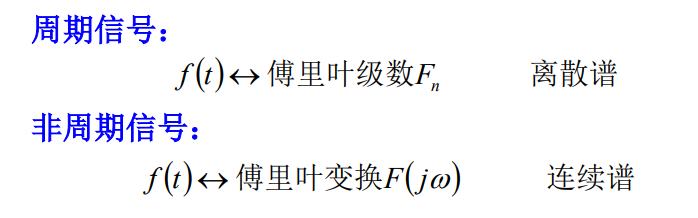正、余弦信号的傅里叶变换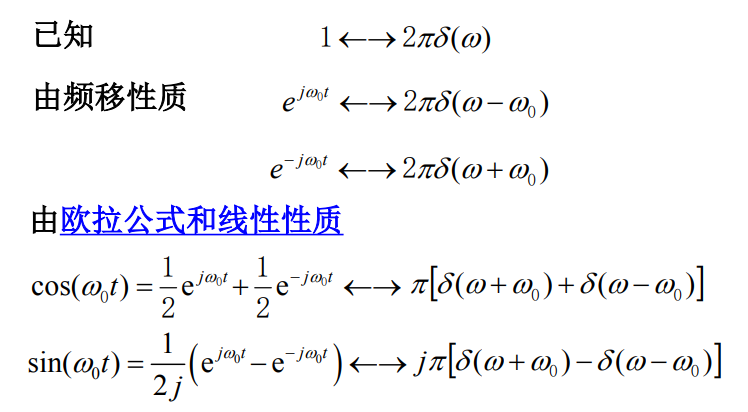一般周期信号的傅里叶变换
周期信号分解为傅里叶级数后再进行傅里叶变换。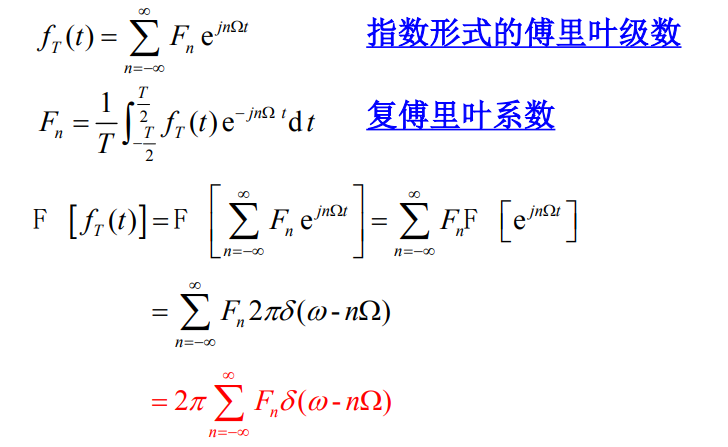Ω

\Omega

为基波频率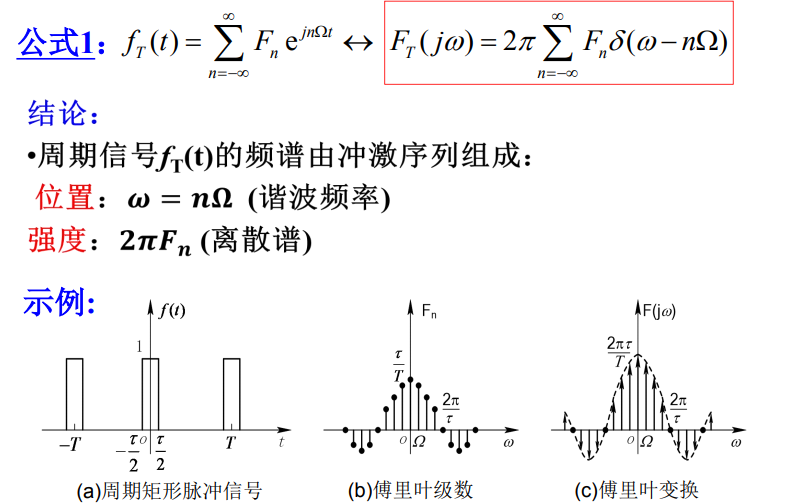傅里叶变换后变成不同频率有相对比例的冲激函数（点变成箭头）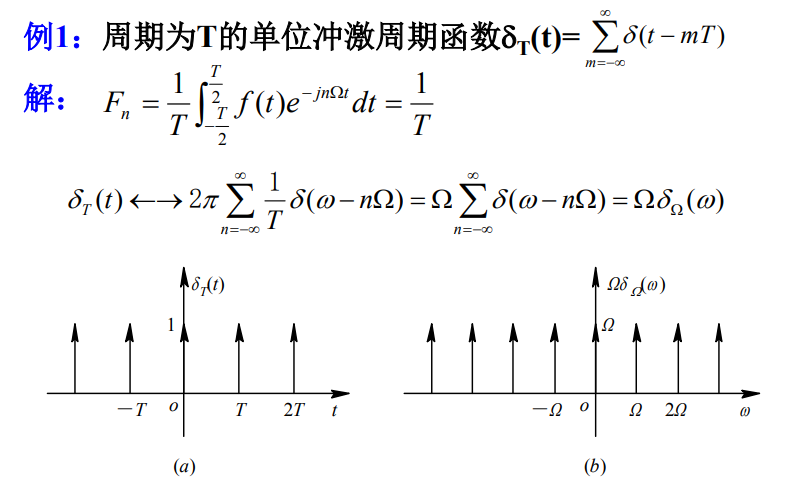2

π

F

n

=

2

π

/

T

=

Ω

2\pi F_n=2\pi/T=\Omega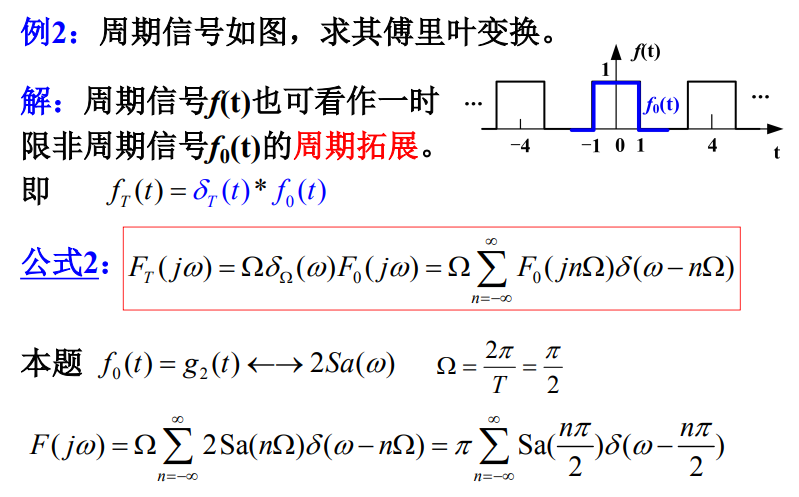时域卷积等于频域乘积
2 周期信号傅里叶级数与傅里叶变换的关系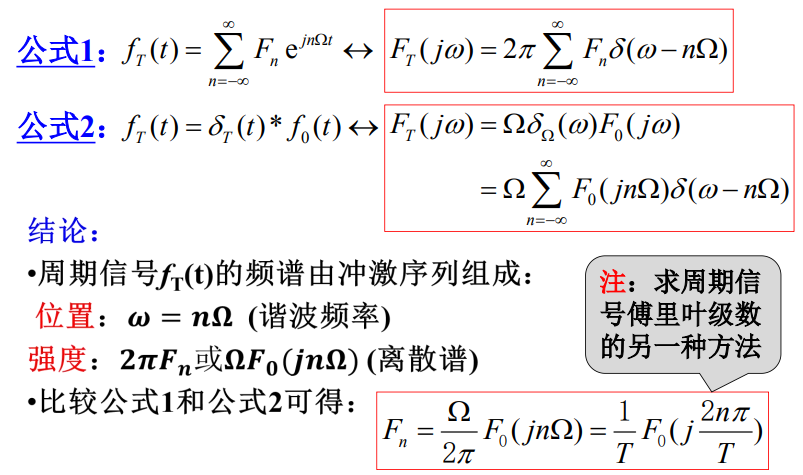由傅里叶变换也可以求傅里叶级数。

《工程信号与系统》作者：郭宝龙等 中国大学MOOC：信号与系统 ，西安电子科技大学，郭宝龙，朱娟娟

展开全文• 信号与系统信号的频谱与傅里叶变换（一图看懂傅里叶变换

Author：AXYZdong 自动化专业 工科男 有一点思考，有一点想法，有一点理性！

文章目录
一图看懂傅里叶变换前言一、周期信号的频谱1、周期信号频谱的相关概念2、周期信号频谱的特点3、谱线的结构与波形参数的关系
二、非周期信号的频谱1、周期信号

→

\to

非周期信号2、频谱密度函数3、傅里叶变换与反变换3.1傅里叶变换3.2傅里叶反变换
4、常用函数的傅里叶变换
总结

一图看懂傅里叶变换从时域来看，我们会看到一个近似为矩形的波，而我们知道这个矩形的波可以被差分为一些正弦波的叠加。 而从频域方向来看，我们就看到了每一个正余弦波的幅值，每两个正弦波之间都还有一条直线，那并不是分割线，而是振幅为 0 的正弦波！ 也就是说，为了组成特殊的曲线，有些正弦波成分是不需要的。随着叠加的递增，所有正弦波中上升的部分逐渐让原本缓慢增加的曲线不断变陡，而所有正弦波中下降的部分又抵消了上升到最高处时继续上升的部分使其变为水平线。一个矩形就这么叠加而成了。但是要多少个正弦波叠加起来才能形成一个标准 90 度角的矩形波呢？不幸的告诉大家，答案是无穷多个。
前言
连续系统频域分析中的 信号的频谱与傅里叶变换
信号的频谱：信号的某种特征量与信号频率变化的关系。
频谱图：将幅度和相位分量用一定高度的直线表示。
一、周期信号的频谱
1、周期信号频谱的相关概念
周期信号频谱：周期信号中各次谐波幅值、相位随频率变化关系。

A

u

∼

ω

A_u\sim \omega

：振幅频谱图

φ

u

∼

ω

\varphi _u\sim \omega

：相位频谱图
三角函数形式分解：

f

(

t

)

=

A

0

2

+

∑

n

=

1

∞

A

n

cos

⁡

(

n

Ω

t

+

φ

n

)

f(t)=\frac{A_0}{2} + \sum_{n=1}^{\infty} A_n \cos(n \Omega t + \varphi_n)

虚指数函数形式分解：

f

(

t

)

=

∑

n

=

−

∞

∞

F

n

e

j

n

Ω

t

f(t)= \sum_{n=-\infty}^{\infty} F_n e^{jn \Omega t}

频谱分类直流分量幅度相位

n

n

单边谱

A

0

2

\frac{A_0}{2}

A

n

A_n

φ

n

\varphi_n

n

=

0

,

1

,

2

,

.

.

.

n=0,1,2,...

双边谱

F

0

F_0

|

F

n

F_n

|

φ

n

\varphi_n

n

=

0

,

±

1

,

±

2

,

.

.

.

n=0, \pm1, \pm2,...

单边谱和双边谱的关系:

cos

⁡

(

n

Ω

t

)

=

1

2

(

e

j

n

Ω

t

+

e

−

j

n

Ω

t

)

F

n

=

∣

F

n

∣

e

j

φ

n

=

1

2

A

n

e

j

φ

n

∣

F

n

∣

=

1

2

A

n

,

φ

n

=

−

arctan

⁡

b

n

a

n

\cos( n \Omega t) = \frac{1}{2} (e^{jn \Omega t}+e^{-jn \Omega t})\\ F_n=|F_n| e^{j \varphi_n} = \frac{1}{2} A_n e^{j \varphi_n}\\ |F_n|= \frac{1}{2} A_n, \varphi_n=- \arctan\frac{b_n}a_n{}

例：周期信号

f

(

t

)

=

1

−

1

2

cos

⁡

(

π

4

t

−

2

π

3

)

+

1

4

sin

⁡

(

π

3

t

−

π

6

)

f(t) = 1- \frac{1}{2} \cos(\frac{\pi}{4}t-\frac{2\pi}{3}) + \frac{1}{4} \sin(\frac{\pi}{3}t-\frac{ \pi}{6})

求该周期信号的基波周期

T

T

，基波角频率

Ω

\Omega

，平均功率

P

P

，并画出它的频谱图。
解：

改

写

f

(

t

)

表

达

式

：

f

(

t

)

=

1

+

1

2

cos

⁡

(

π

4

t

+

π

3

)

+

1

4

cos

⁡

(

π

3

t

−

2

π

3

)

改写f(t)表达式：f(t)=1 + \frac{1}{2} \cos(\frac{\pi}{4}t + \frac{\pi}{3}) + \frac{1}{4} \cos(\frac{\pi}{3}t-\frac{ 2\pi}{3})

1

2

cos

⁡

(

π

4

t

+

π

3

)

周

期

T

1

=

8

\frac{1}{2} \cos(\frac{\pi}{4}t + \frac{\pi}{3}) 周期 T_1=8

1

4

cos

⁡

(

π

3

t

−

2

π

3

)

周

期

T

2

=

6

\frac{1}{4} \cos(\frac{\pi}{3}t-\frac{ 2\pi}{3})周期 T_2=6

∴

f

(

t

)

周

期

T

=

24

,

基

波

角

频

率

Ω

=

2

π

T

=

π

12

\therefore f(t) 周期T=24, 基波角频率 \Omega = \frac{ 2\pi}{T} =\frac{ \pi}{12}

由

帕

斯

瓦

尔

等

式

，

P

=

1

+

1

2

⋅

(

1

2

)

2

+

1

2

⋅

(

1

4

)

2

=

37

32

由帕斯瓦尔等式，P=1+\frac{ 1}{2} \cdot(\frac{ 1}{2})^2 +\frac{ 1}{2} \cdot(\frac{ 1}{4})^2 =\frac{ 37}{32}

频谱图：

1

2

cos

⁡

(

π

4

t

+

π

3

)

\frac{1}{2} \cos(\frac{\pi}{4}t + \frac{\pi}{3})

是

f

(

t

)

f(t)

的

[

π

/

4

]

/

[

π

/

12

]

=

3

[\pi/4]/[\pi/12]=3

次谐波分量；

1

4

cos

⁡

(

π

3

t

−

2

π

3

)

\frac{1}{4} \cos(\frac{\pi}{3}t-\frac{ 2\pi}{3})

是

f

(

t

)

f(t)

的

[

π

/

3

]

/

[

π

/

12

]

=

4

[\pi/3]/[\pi/12]=4

次谐波分量；2、周期信号频谱的特点
1、离散型：以基频

Ω

\Omega

为间隔的若干离散谱线组成  2、谐波性：谱线仅含有基频

Ω

\Omega

的整数倍分量  3、收敛性：整体趋势减小
周期信号频谱的特点简要的概括了一下
3、谱线的结构与波形参数的关系
1、

T

T

一定，

τ

\tau

变小，此时

Ω

\Omega

（谱线间隔）不变。两零点之间的谱线数：

ω

Ω

=

2

π

τ

/

2

π

T

,

增

多

\frac{\omega}{\Omega} = \frac{2 \pi}{\tau} /\frac{2\pi}{T},增多

2、

τ

\tau

一定，

T

T

增大，间隔

Ω

\Omega

减小，频谱变密，幅度减小。
如果周期

T

T

无限增长（

T

→

∞

T\to \infty

），周期信号就变成了非周期信号，那么，谱线间隔将趋于零，周期信号的离散频谱就过渡到非周期信号的连续频谱。各频率分量的幅度也趋近于无穷小。
二、非周期信号的频谱
1、周期信号

→

\to

非周期信号
频谱函数：

F

n

=

1

T

∫

−

2

T

2

T

f

(

t

)

e

−

j

n

Ω

t

d

t

F_n= \frac{1}{T} \int_{-\frac{2}{T}}^{ \frac{2}{T}} f(t) e^{-j n \Omega t}dt

T

→

∞

T\to \infty

时：

f

(

t

)

周

期

信

号

→

非

周

期

信

号

F

n

→

0

谱

线

间

隔

Ω

→

0

离

散

频

谱

→

连

续

频

谱

，

频

谱

幅

度

→

0

f(t) 周期信号\to 非周期信号\\ F_n \to0\\ 谱线间隔 \Omega \to 0\\ 离散频谱\to 连续频谱 ，频谱幅度\to 0

2、频谱密度函数
频谱函数：

F

n

=

1

T

∫

−

2

T

2

T

f

(

t

)

e

−

j

n

Ω

t

d

t

F_n= \frac{1}{T} \int_{-\frac{2}{T}}^{ \frac{2}{T}} f(t) e^{-j n \Omega t}dt

T

→

∞

T\to \infty

时：

Ω

→

d

ω

(

无

穷

小

量

)

n

Ω

→

ω

(

离

散

→

连

续

)

\Omega \to d\omega (无穷小量)\\ n\Omega \to \omega (离散\to连续)\\

F

(

j

ω

)

=

lim

⁡

T

→

∞

F

n

1

/

T

=

lim

⁡

T

→

∞

F

n

T

F(j \omega)=\lim_{T\to \infty} \frac{F_n}{1/T}=\lim_{T\to \infty}F_nT

=

lim

⁡

T

→

∞

∫

−

2

T

2

T

f

(

t

)

e

−

j

n

Ω

t

d

t

=\lim_{T\to \infty} \int_{-\frac{2}{T}}^{\frac{2}{T}} f(t) e^{-j n \Omega t}dt

=

∫

−

∞

∞

f

(

t

)

e

−

j

ω

t

d

t

=\int_{-\infty}^{\infty} f(t) e^{-j \omega t}dt

3、傅里叶变换与反变换
3.1傅里叶变换

F

(

j

ω

)

=

∫

−

∞

∞

f

(

t

)

e

−

j

ω

t

d

t

F(j \omega)= \int_{-\infty}^{\infty} f(t) e^{-j \omega t}dt

F

(

j

ω

)

F(j \omega)

称为

f

(

t

)

f(t)

的傅里叶变换

F

(

j

ω

)

F(j \omega)

一般为复数，写成

F

(

j

ω

)

F(j \omega)

=

∣

F

(

j

ω

)

∣

e

j

φ

(

ω

)

|F(j \omega)| e^{j \varphi(\omega)}

F

(

j

ω

)

∼

ω

F(j \omega)\sim \omega

：幅频度谱图，频率

ω

\omega

的偶函数

φ

u

∼

ω

\varphi _u\sim \omega

：相位频谱图，频率

ω

\omega

的奇函数
3.2傅里叶反变换

f

(

t

)

=

1

2

π

∫

−

∞

∞

F

(

j

ω

)

e

j

ω

t

d

ω

f(t)= \frac{1}{2\pi}\int_{-\infty}^{\infty}F(j\omega)e^{j \omega t}d\omega

符号差别：4、常用函数的傅里叶变换

e

−

α

t

ϵ

(

t

)

⟷

1

α

+

j

ω

e^{- \alpha t}\epsilon(t) \longleftrightarrow \frac{1}{\alpha+j \omega}

e

−

α

∣

t

∣

⟷

2

α

α

2

+

ω

2

e^{- \alpha |t|} \longleftrightarrow \frac{2\alpha}{\alpha ^2+ \omega ^2}

g

τ

(

t

)

⟷

τ

S

a

(

ω

τ

2

)

g_\tau(t) \longleftrightarrow \tau Sa(\frac{\omega \tau}{2})

δ

(

t

)

⟷

1

\delta(t) \longleftrightarrow 1

δ

′

(

t

)

⟷

j

ω

\delta'(t) \longleftrightarrow j \omega

1

⟷

2

π

δ

(

ω

)

1 \longleftrightarrow2\pi \delta(\omega)

s

g

n

(

t

)

⟷

2

j

ω

sgn(t) \longleftrightarrow \frac{2}{ j \omega}

ϵ

(

t

)

⟷

π

δ

(

ω

)

+

1

j

ω

\epsilon(t) \longleftrightarrow \pi \delta(\omega) + \frac{1}{ j \omega}

总结时域里面原函数

⟶

\longrightarrow

频域里面相函数 频域里面相函数

⟶

\longrightarrow

时域里面原函数
周期信号

→

\to

傅里叶级数

→

\to

频谱
非周期信号

→

\to

傅里叶变换

→

\to

频谱

「你可能还想看」系列文章： 【信号与系统】笔记合集，你确定不收藏吗？我已经收藏了
如果觉着帮到你的话，点个赞支持一下呢！！！^ _ ^ 码字不易，大家的支持就是我坚持下去的动力。点赞后不要忘了关注我哦！

说明：部分图片来源于网络，如有侵权请联系我删除。

展开全文人工智能 jrebel webgl cstring
• 信号的单双边谱及其特点进行了讲解，重点探讨了LTI系统频域分析所使用的傅里叶变换/级数分析法。
信号分解、傅里叶变换与信号谱

在【信号与系统|吴大正】4：信号分解、傅里叶变换与信号谱(上)中我们着重讨论了信号的分解，以及从傅里叶级数引出傅里叶变换的定义及相关性质；  在这篇文章中，我们开始讨论信号的不同频谱及其特点，以及将傅里叶引入到信号与系统的分析过程中

信号谱与谱估计
能量谱
1. 信号能量的定义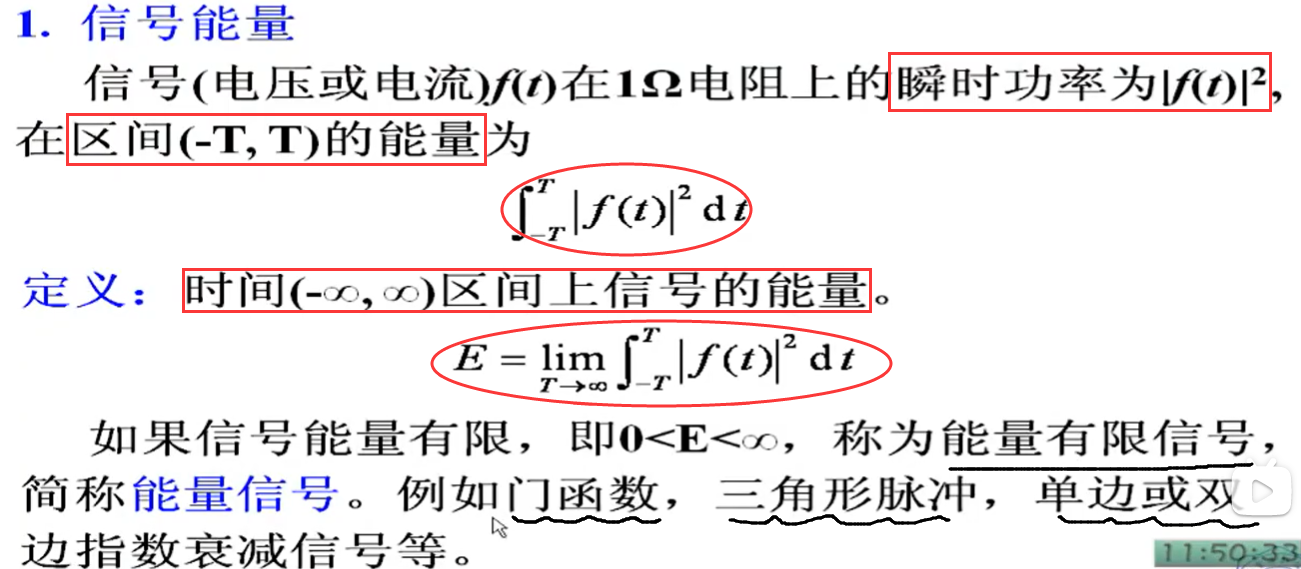2. 帕斯瓦尔方程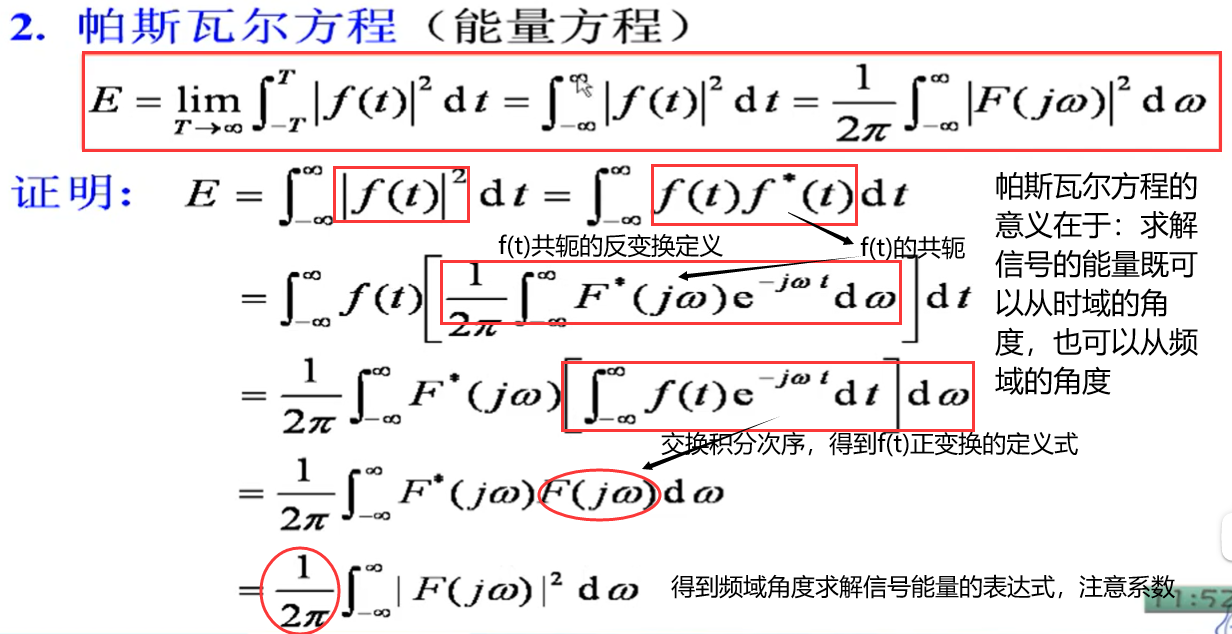3. 能量密度谱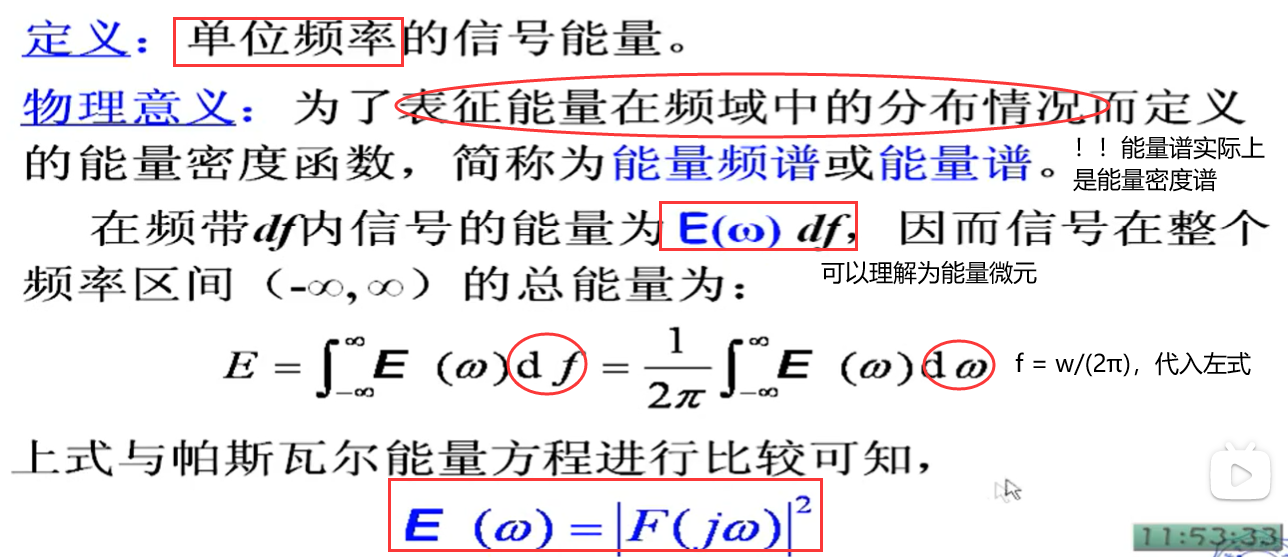能量有限信号的自相关函数和其能量密度谱是一对傅里叶变换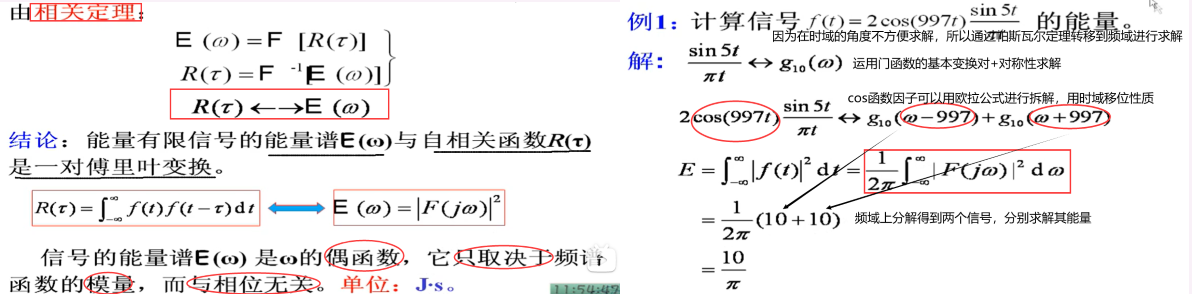功率谱
1. 信号的功率、功率谱与功率密度谱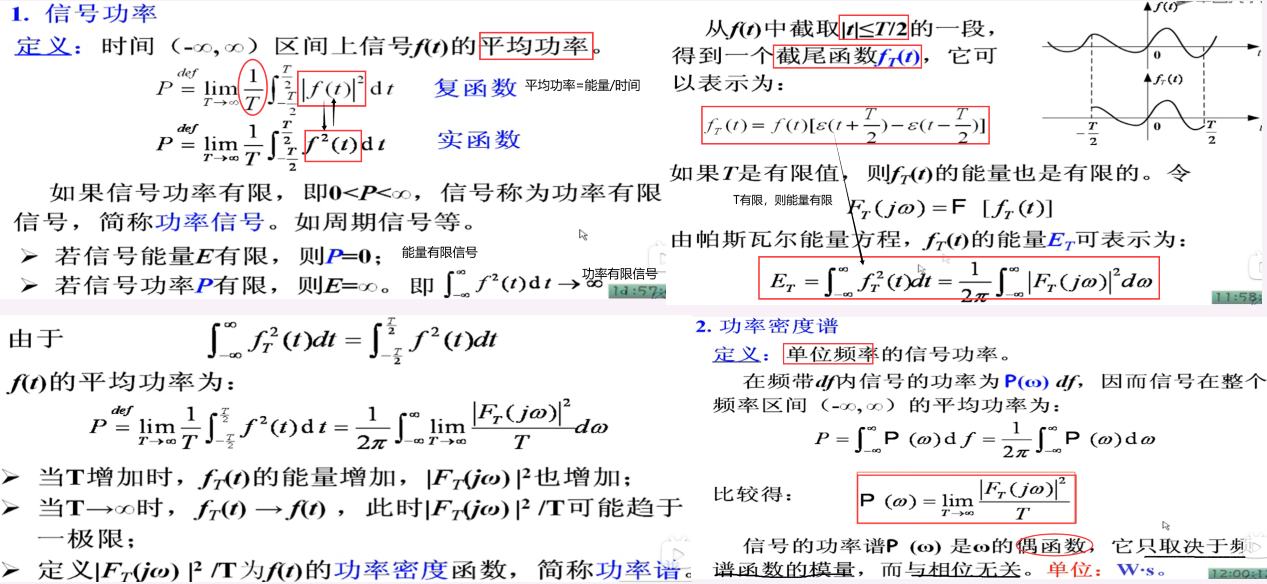2. 自相关函数与功率密度谱
功率有限信号的自相关函数和其功率谱构成傅里叶变换对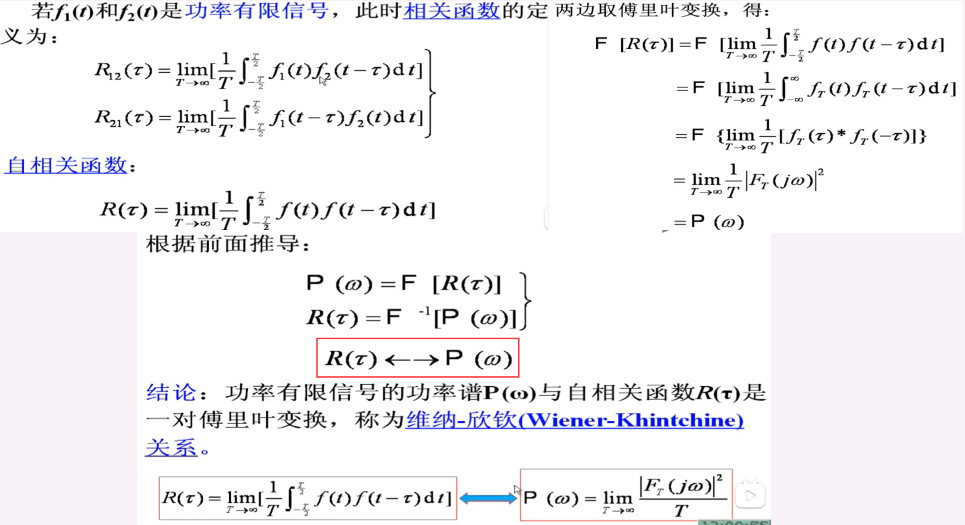白噪声功率谱密度的估计

白噪声属于随机信号，是一种没有确定的频谱，但是有相关统计量的一种信号，因此不能直接用频谱图对白噪声信号进行表示。 根据前文讨论过的信号功率谱与能量谱，我们也可以利用白噪声信号的自相关函数以及傅里叶变换，求出其功率谱密度，借助功率谱来描述其频域特性。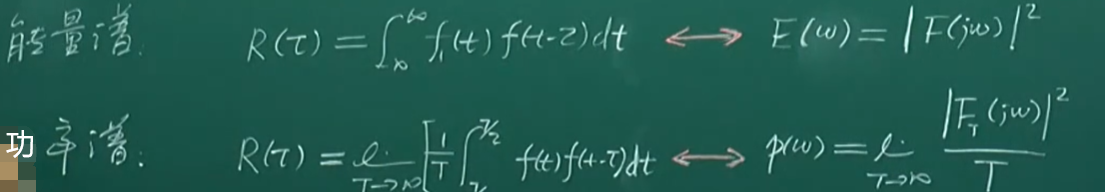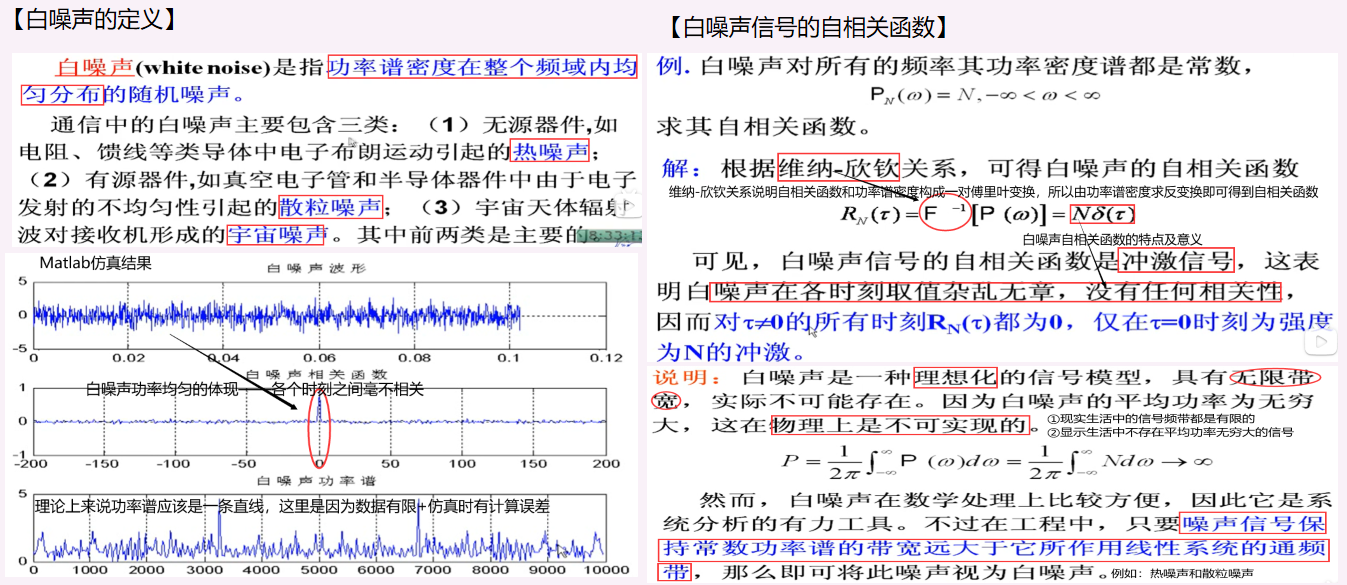傅里叶变换与系统分析
周期信号与傅里叶变换

周期信号的傅里叶变换周期信号的傅里叶级数与傅里叶变换

1. 周期信号的傅里叶变换

按照我们目前所讨论的知识点，对于周期信号我们可以用傅里叶级数进行展开，对于非周期信号我们可以用傅里叶变换进行求解； 于是我们开始思考是否可以对周期信号也进行傅里叶变换的作用，这样就可以让傅里叶变换在周期/非周期信号领域进行统一。  故：我们的核心就是要在傅里叶级数与傅里叶变换之间寻找关系，已知傅里叶级数是若干三角函数项(或虚指数项)的总和，所以我们先来探究三角函数（虚指数函数）的傅里叶变换结果。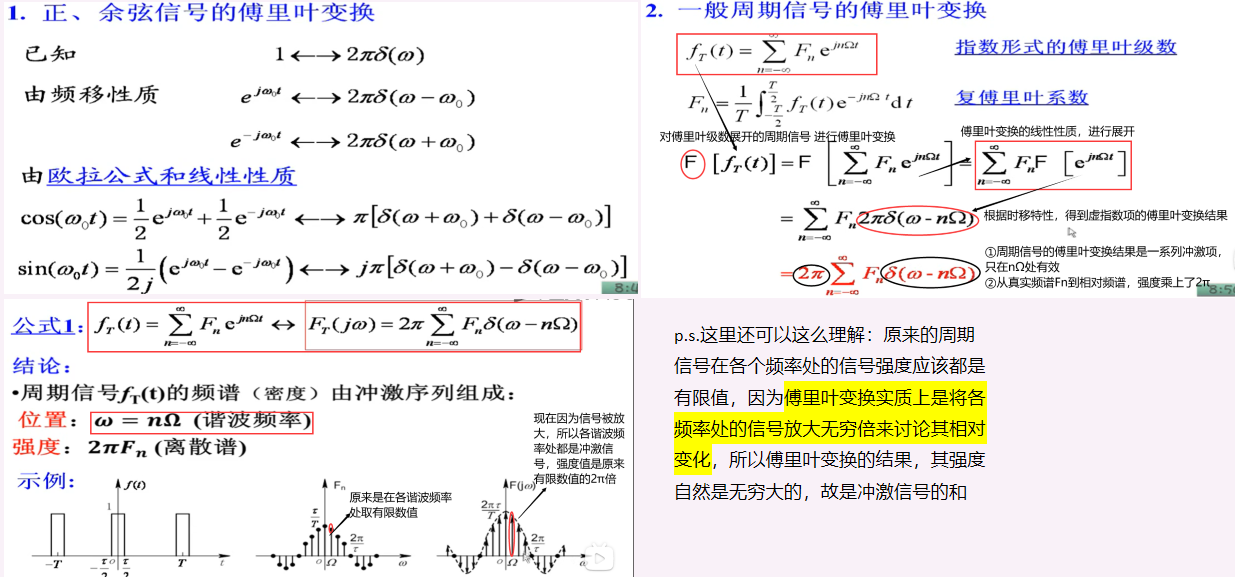2. 方法论——求解周期信号的傅里叶变换
展开成傅里叶级数，得到傅里叶系数，利用公式结论将周期信号分解成基本信号与冲激序列信号的卷积，利用傅里叶变换的卷积定理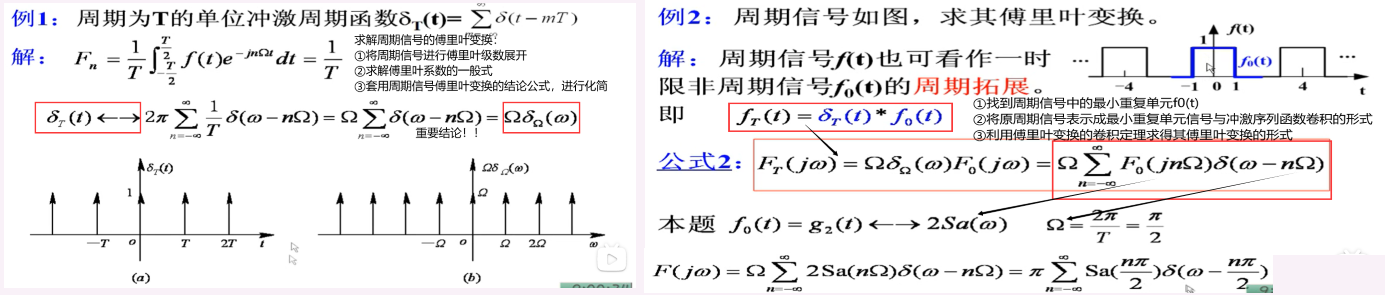3. 傅里叶级数与傅里叶变换之间的关系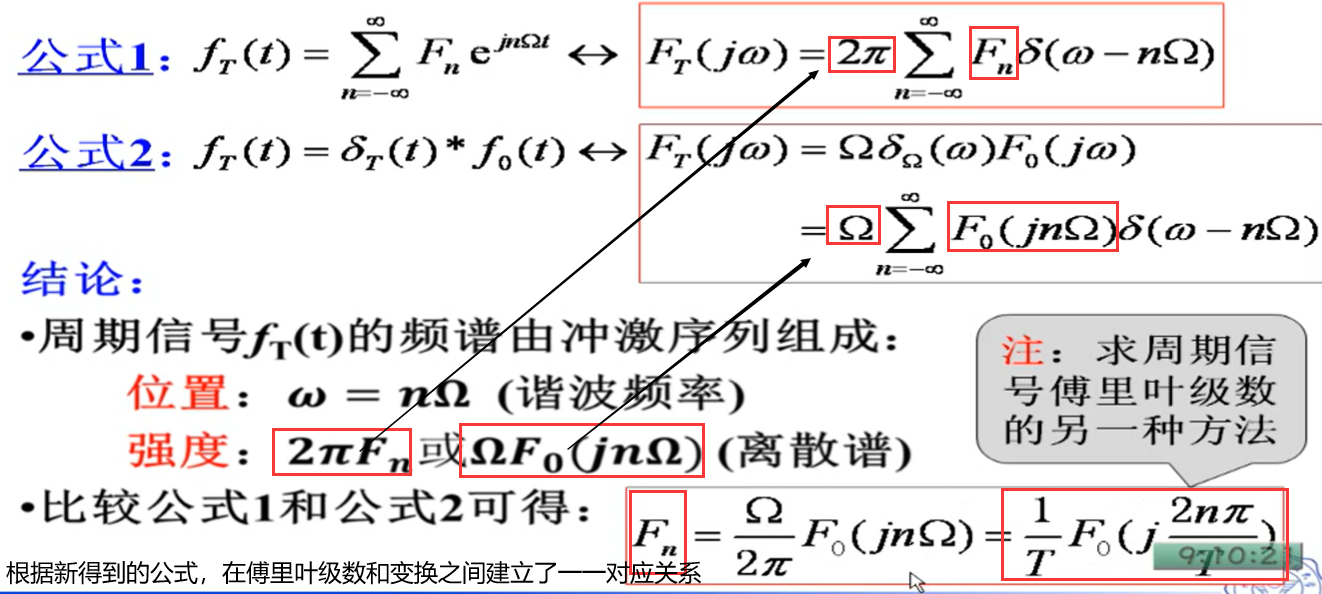信号、系统与响应

基本信号作用于LTI系统的响应一般信号作用于LTI系统的响应

1. 基本信号作用于LTI系统的响应
基本信号是虚指数信号ejwt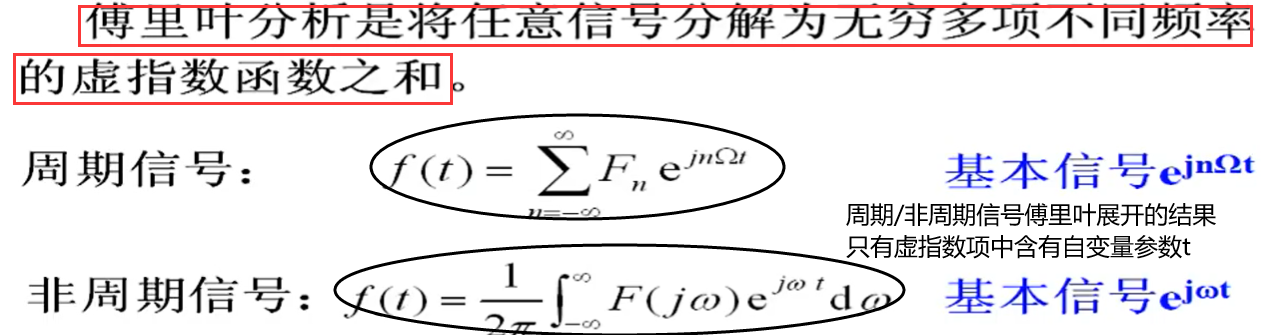所求响应为零状态响应，也即全响应

在频域分析中，基本信号的定义域设置为(-∞，+∞)，而t=-∞的时候总可以认为系统的状态为0，所以这里求解的全响应也就是零状态响应。

y(t) = H(jω)·ejωt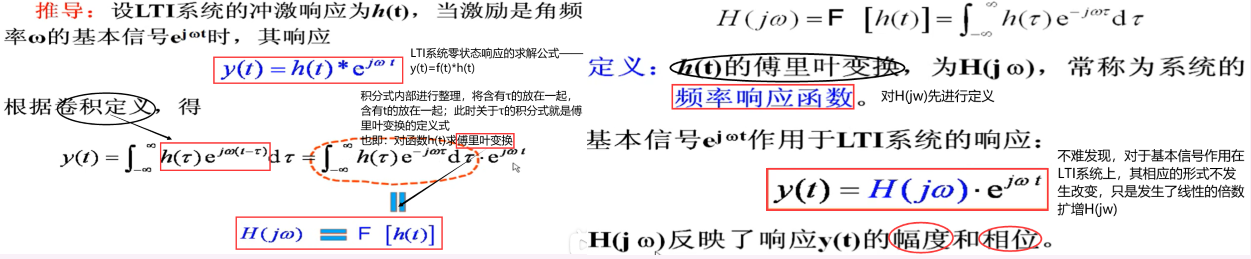2. 一般信号作用于LTI系统的响应

要对一般信号进行分解，然后找到其和基本信号的关系，在找到它们响应之间的关系式。

推导过程

利用了傅里叶变换的定义式，以及LTI系统零状态响应的齐次性和可加性； ①明确基本信号响应的特点——基本信号的响应依然是基本信号，只不过进行了线性的缩放 ②任意信号的分解实际上就是傅里叶级数的分解，将任意信号展开成基本信号的线性组合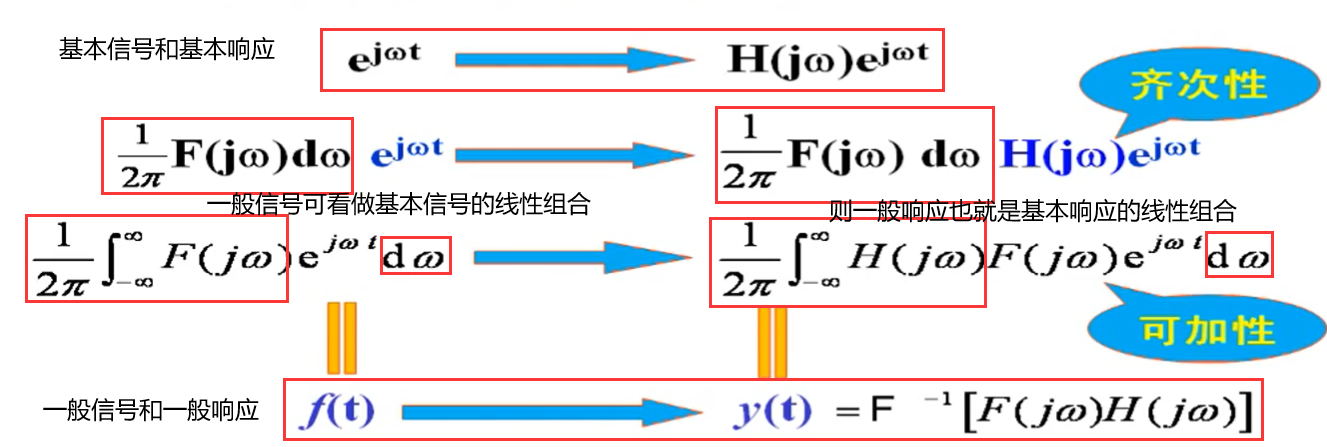结论

LTI系统零状态响应的求解式子y(t) = f(t)*h(t)是一直成立的； 只不过在频域分析中，信号要通过傅里叶变换得到频域表现形式，而根据时域和频域的转换规则，卷积运算也相应变成乘积运算，得到Y(jω)=F(jω)·H(jω)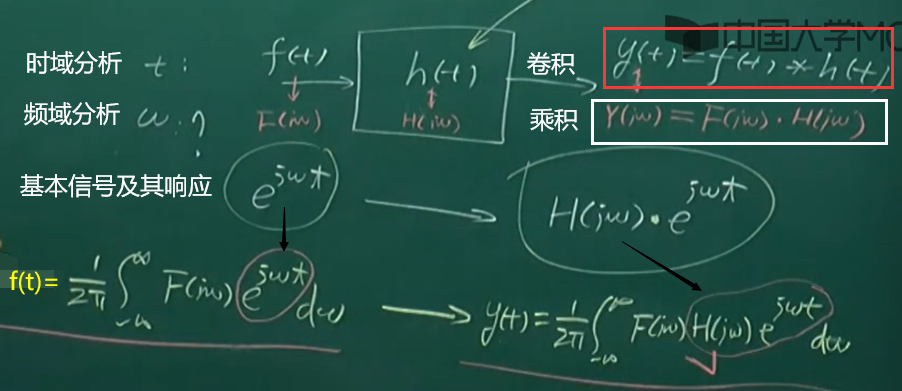傅里叶分析法

傅里叶变换分析法傅里叶级数分析法

1. 傅里叶变换分析法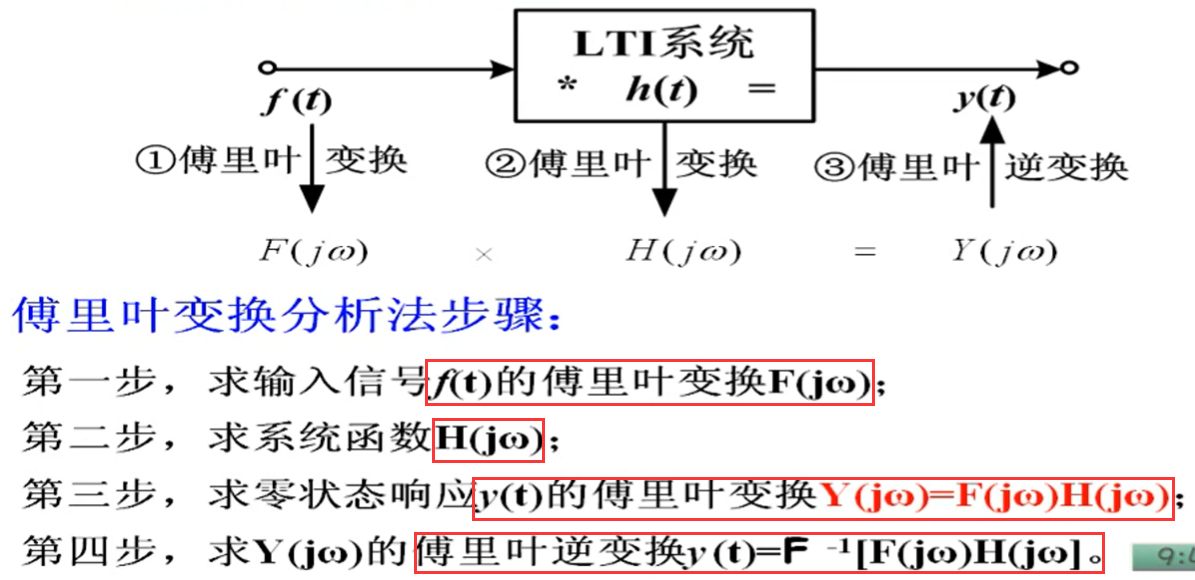由上图所示，因为从时域转换成频域，使得卷积运算变成乘法运算缩减了卷积运算的计算量，但同时也增加了信号之间进行傅里叶正反变换的次数。
2. 傅里叶级数分析法

傅里叶变换分析和傅里叶级数都是在进行系统分析时的可选选择，两者并不矛盾。 傅里叶变换分析对周期/非周期均可使用；傅里叶级数分析只能对周期信号使用。

（1）复指数级数的分析
推导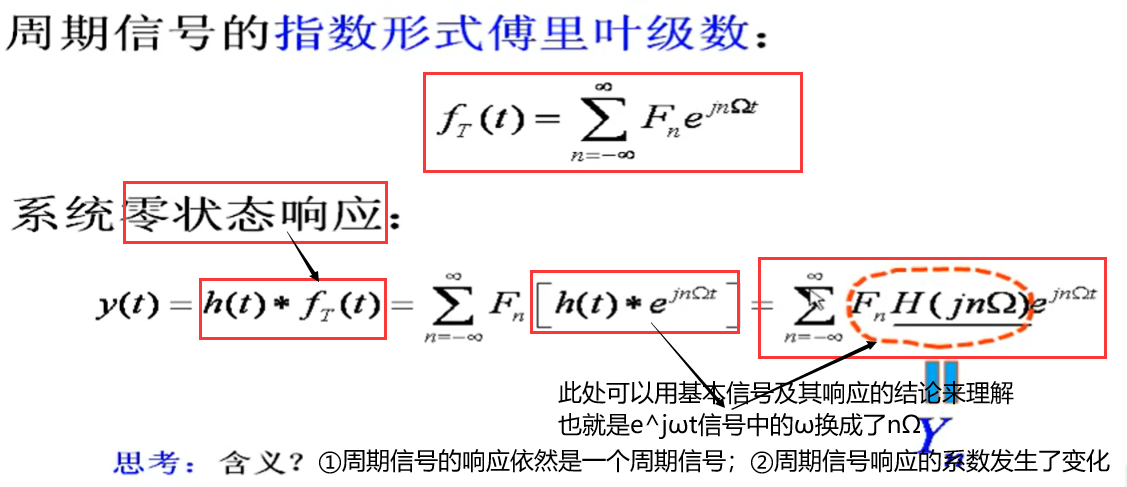方法论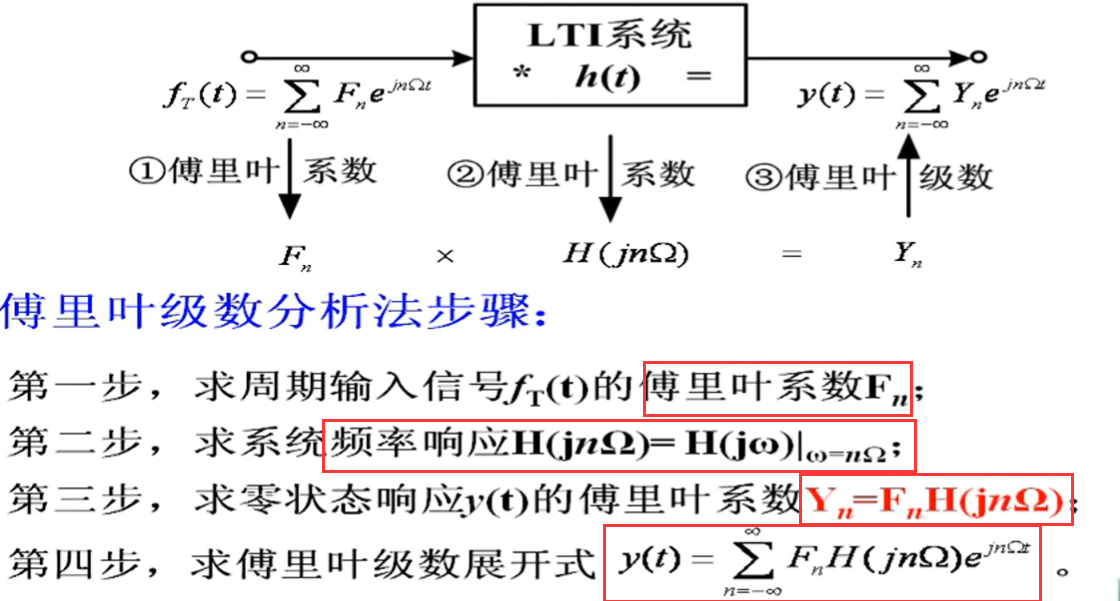（2）三角级数的分析  可以利用欧拉公式将三角级数转换成复指数形式，利用上式的结论  也可以直接对三角函数的响应结构进行讨论，以下仅给出结论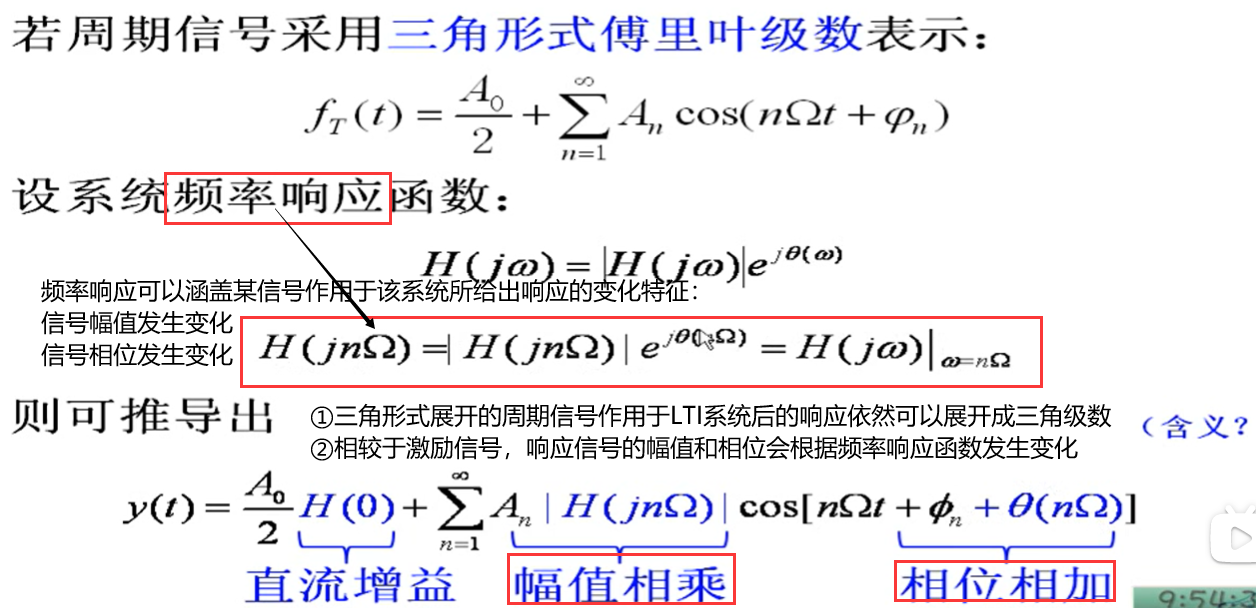3. LTI系统频域分析示例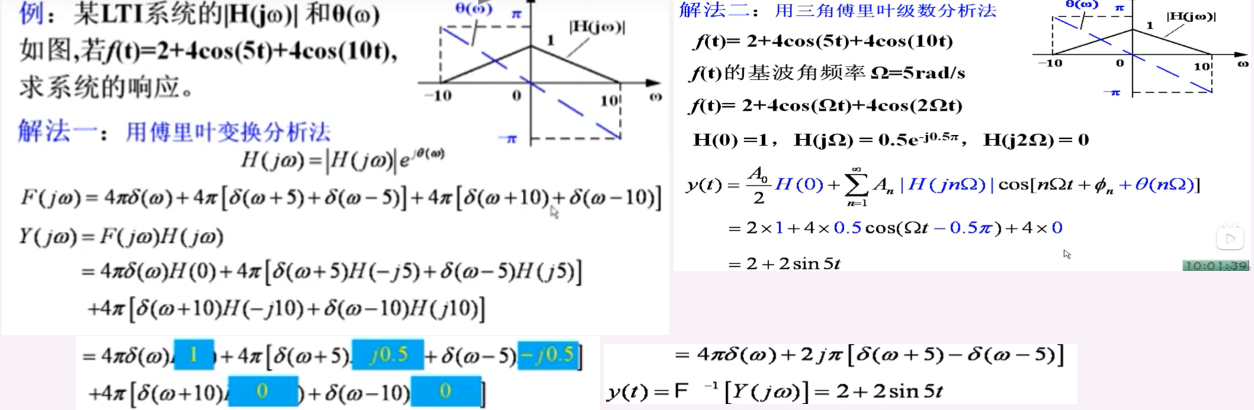展开全文卷积
• 信号分解、傅里叶变换与信号信号的分解 在学习【信号分解】这一部分时，脑海里要有两个概念： 其一，我们整本书学习的思路就是围绕着将信号分解成基本信号，将系统的响应转变成基本响应这一思路来开展的； 其二...卷积
• 对于所有涉及到电子、通信、控制等等专业的人来说，傅里叶变换是绕... 傅里叶变换的作用是将原来难以处理的时域信号转换为易于分析的频域信号，也就是信号的频谱。在频域进行处理和加工之后，还可以利用傅里叶反变换将
• 傅里叶变换、拉普拉斯变换、Z变换公式和性质表格汇总。傅里叶变换、拉普拉斯变换、Z变换公式和性质表格汇总。傅里叶变换、拉普拉斯变换、Z变换公式和性质表格汇总。傅里叶变换、拉普拉斯变换、Z变换公式和性质表格...
• 理论上只有满足绝对可和条件的非周期信号才能傅里叶变换，而周期信号（不绝对可和）不满足绝对可和条件的非周期信号无法进行傅里叶变换。 实际中因为正余弦周期信号都有傅里叶变换，普通周期信号可以先求傅里叶...线性代数
•技术
• 傅里叶变换的离散型周期性 文章目录傅里叶变换的离散型周期性前言1.连续时间连续频率2.连续时间离散频率3.离散时间连续频率4.离散时间离散频率 前言 通过傅里叶级数，即周期函数可以转换为一系列离散...dft
• 信号处理领域，存在诸多变换，比如标题中的五个变换。本文将对这五个变换进行介绍和比较。在开始之前，我们需要先理清什么是...通常傅里叶变换只适合处理平稳信号，对于非平稳信号，由于频率特性会随时间变化，为了matlab 小波分析
• 信号与系统 第二章傅里叶变换 包括傅里叶级数（指数和三角形式），傅里叶变换，典型信号傅里叶变换傅里叶变换性质和采样定理
• 信号与系统, 考研常用变换对及性质梳理, 傅里叶变换(Fourier Transform, FT), 拉普拉斯变换(Laplace Transform, LT), z变换(z Transform, ZT), 081000信息通信工程, 081002信号与信息处理, 081001通信信息系统, ...
• 通过傅里叶级数展开，将周期信号分解为一系列...他们的关系在信号与系统（11）有所分析，总结如下表所示： 相关对比项 傅里叶级数 傅里叶变换 对原信号f(t)f(t)f(t)的分解 f(t)=∑n=−∞+∞[Cn⋅ej(nΩt)]
• 应用web和matlab的《信号与系统基础教程》 傅里叶级数与傅里叶变换 可以想出来，傅里叶级数是傅里叶变换的一个特例罢了，同时傅里叶级数通常针对的周期函数，而傅里叶变换则针非周期的函数（满足地狄更斯积分条件的...
• 实验四 傅里叶变换系统的频域分析，实验代码，实验报告
• 当引入广义函数的概念后，许多不满足绝对可积条件的函数也能进行傅里叶变换，这给信号与系统分析带来很大方便。 一、奇异函数的傅里叶变换 1、冲激函数的频谱 方法一：根据傅里叶变换的定义式，并且考虑到冲激函数的...
• 傅里叶变换变换的性质揭示了信号的时域特性和频域特性之间的内在联系。讨论傅里叶变换的性质，目的在于： •了解时频域特性的内在联系； • 利用性质求F(jω)F(jω)F(jω)； •了解在通信系统领域中的应用。 1 线性...
• 点击上方蓝字关注“公众号”MATLAB傅里叶变换系统的频域分析当初学信号与系统，天书睡过去啊，一到考试心中mmp。理论的枯燥，有了MATLAB就生动形象啦。 本节旨在掌握：1、学会用MATLAB实现连续时间信号傅里叶变换2...
• 文章目录非周期信号的频谱--傅里叶变换1 频谱密度函数1.1 引出1.2 频谱密度函数2 傅里叶变换2.1 傅里叶变换2.2 傅里叶反变换2.3 傅里叶变换对2.4 说明3 常用函数的傅里叶变换3.1 单边指数函数3.2 双边指数函数 ...
• 单边指数信号 我们设单边指数信号的表达式为 其中 为正实数。则 得 双边指数信号 设双边指数信号的表达式为
• 信号与系统第11讲-从离散周期信号傅里叶级数到离散时间傅里叶变换.ppt
• 常用的连续傅里叶变换对及其对偶关系 连续傅里叶变换性质及其对偶关系...